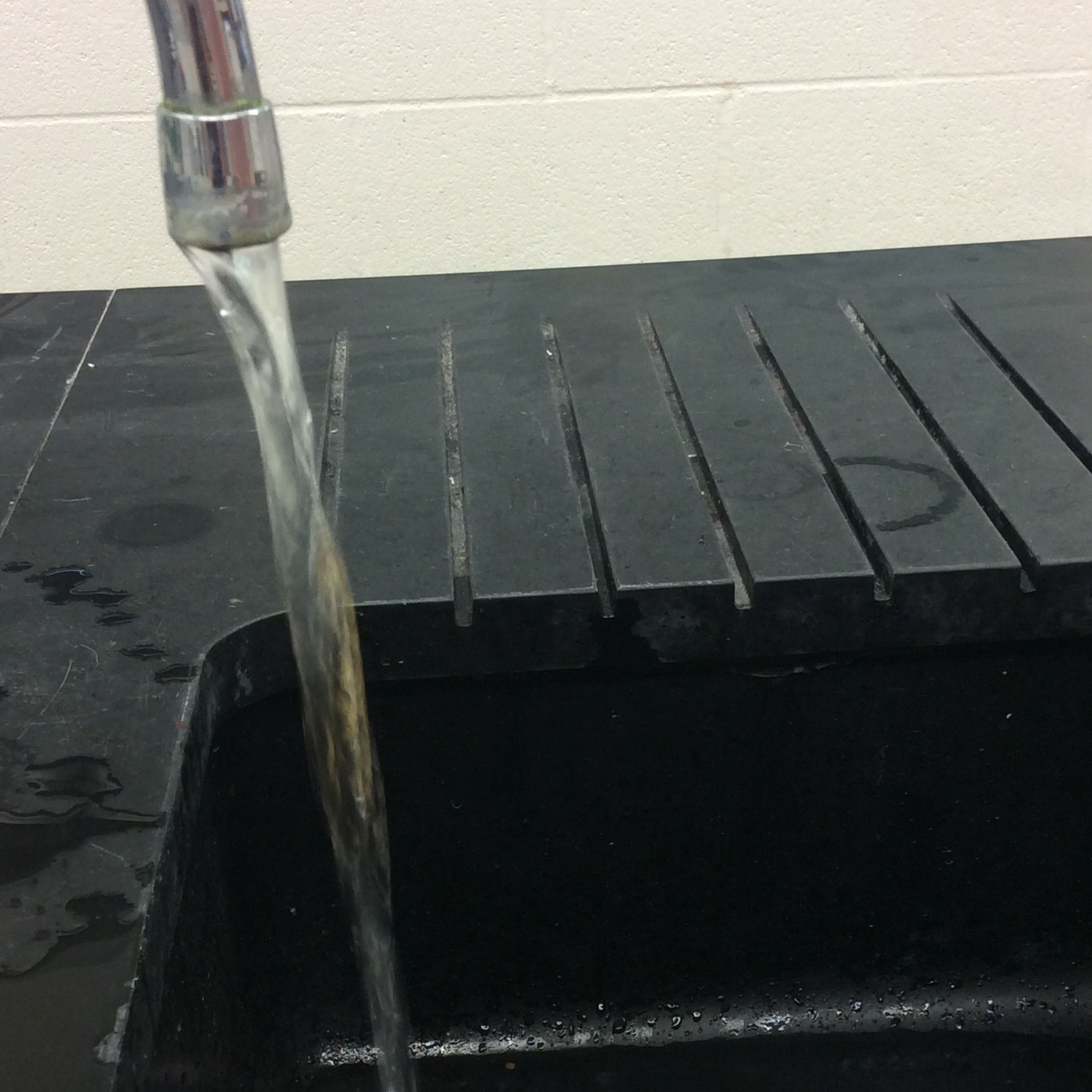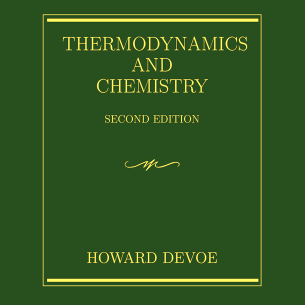A state of chemical equilibrium is reached when the concentration of reactants and product are constant over time (Wikipedia). This often occurs when the forward and backwards reaction occur at the same rate.

In contrast, steady state is when the state variables are constant over time while there is a flow through the system (Wikipedia). The state variables parameters that depend on the current state of a system, not on how the system got to that state.

Thermal equilibrium is reached when two objects in contact with each other reach the same temperature, and are therefor not exchanging heat (Wikipedia). For example, if a gallon of milk is taken out of a cold fridge and placed on the counter, the counter and the milk are at thermal equilibrium when they reach the same temperature.

An example of steady state can be found a bathtub with the drain open and water being added. If water is leaving the tub at the same rate that water is being added, the state variable volume will be constant over time.

The following video takes the bathtub example to a larger scale to demonstrate the difference between equilibrium and steady state.

##Online Textbooks

Need more help? These thermodynamic textbooks are free to view and entirely online!

Visit Page »